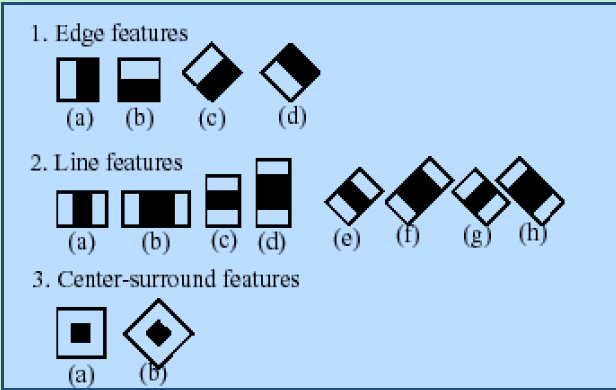# opencv5-objdetect之级联分类器

1、先设定不同的分类器：

 string face_cascade_name = "haarcascade_frontalface_alt.xml";
string eyes_cascade_name = "haarcascade_eye_tree_eyeglasses.xml";
2、然后进行分类器类的初始化：

CascadeClassifier face_cascade;
CascadeClassifier eyes_cascade;
 if( !face_cascade.load( face_cascade_name ) ){ printf("--(!)Error loading\n"); return -1; };
if( !eyes_cascade.load( eyes_cascade_name ) ){ printf("--(!)Error loading\n"); return -1; };

3、进行对象的检测

void CascadeClassifier::detectMultiScale(const Mat& image, vector<Rect>& objects, double scaleFactor=1.1, int minNeighbors=3, int flags=0,
Size minSize=Size(), Size maxSize=Size())

vector<Rect> faces;
Mat detFrame_gray;

cvtColor( srcFrame, detFrame_gray, CV_BGR2GRAY );
equalizeHist( detFrame_gray, detFrame_gray );
face_cascade.detectMultiScale( detFrame_gray, faces, 1.1, 2, 0|CV_HAAR_SCALE_IMAGE, Size(30, 30) );

 for( int i = 0; i < faces.size(); i++ )
{
Point center( faces[i].x + faces[i].width*0.5, faces[i].y + faces[i].height*0.5 );
ellipse( frame, center, Size( faces[i].width*0.5, faces[i].height*0.5), 0, 0, 360, Scalar( 255, 0, 255 ), 4, 8, 0 );

Mat faceROI = frame_gray( faces[i] );
vector<Rect> eyes;

//-- 在每张人脸上检测双眼
eyes_cascade.detectMultiScale( faceROI, eyes, 1.1, 2, 0 |CV_HAAR_SCALE_IMAGE, Size(30, 30) );

for( int j = 0; j < eyes.size(); j++ )
{
Point center( faces[i].x + eyes[j].x + eyes[j].width*0.5, faces[i].y + eyes[j].y + eyes[j].height*0.5 );
int radius = cvRound( (eyes[j].width + eyes[i].height)*0.25 );
circle( frame, center, radius, Scalar( 255, 0, 0 ), 4, 8, 0 );
}
}

notes：不论是上面的人脸还是眼睛检测，其中的第一个参数都是被检测的图像，而且在后面的画椭圆和圆的过程中第一个参数仍然是原图像，最后加个imshow（）的函数就可以进行显示检测到的结果。posted @ 2015-01-01 10:49  仙守  阅读(2439)  评论(0编辑  收藏  举报